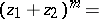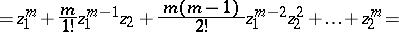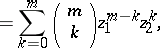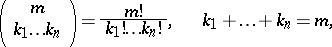# Newton binomial

(diff) ← Older revision | Latest revision (diff) | Newer revision → (diff)

binomium of Newton

The formula for the expansion of an arbitrary positive integral power of a binomial in a polynomial arranged in powers of one of the terms of the binomial:(*)whereare the binomial coefficients. Forterms formula (*) takes the formFor an arbitrary exponent, real or even complex, the right-hand side of (*) is, generally speaking, a binomial series.

The gradual mastering of binomial formulas, beginning with the simplest special cases (formulas for the "square" and the "cube of a sum" ) can be traced back to the 11th century. I. Newton's contribution, strictly speaking, lies in the discovery of the binomial series.